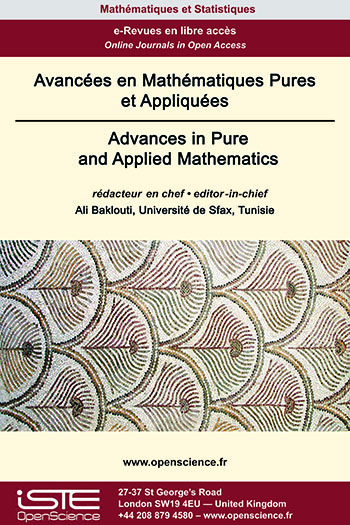# Vol 14 - Issue 3 (June 2023)

## List of Articles

Global existence of solutions to the spherically symmetric Einstein-Vlasov-Maxwell system
R. Moutngui, P. Noundjeu

We prove that the initial value problem with small data for the asymptotically flat spherically symmetric Einstein-Vlasov-Maxwell system admits the global in time solution in the case of the non zero shift vector. This result extends the one already known for chargeless case.

First Chen Inequality for General Warped Product Submanifolds of a Riemannian Space Form and Applications
Abdulqader MUSTAFA, Cenap OZEL, Alexander PIGAZZINI, Ramandeep KAUR, Gauree SHANKER

In this paper, the first Chen inequality is proved for general warped product submanifolds in Riemannian space forms, this inequality involves intrinsic invariants (δ-invariant and sectional curvature) controlled by an extrinsic one (the mean curvature vector), which provides an answer for Chen’s Problem 1 relating to establish simple relationships between the main extrinsic invariants and the main intrinsic invariants of a submanifold. As a geometric application, this inequality is applied to derive a necessary condition for the immersed submanifold to be minimal in Riemannian space forms, which presents a partial answer for the well-known problem proposed by S.S. Chern, Problem 2. For further research directions, we address a couple of open problems; namely Problem 3 and Problem 4.

Characterization of some closed linear subspaces of Morrey spaces and approximation
Nouffou Diarra, Ibrahim Fofana

Let $1\leq q\leq\alpha < \infty. \left\{(L^{q}, l^{p})^{\alpha}(\mathbb{R}^d):\alpha\leq p\leq\infty \right\}$ is a nondecreasing family of Banach spaces such that the Lebesgue space is $L^{\alpha}(\mathbb{R}^d)$ its minimal element and the classical Morrey space $\mathcal{M}_{q}^{\alpha}(\mathbb{R}^d)$ is its maximal element. In this note we investigate some closed linear subspaces of $(L^{q}, l^{p})^{\alpha}(\mathbb{R}^d)$. We give a characterization of the closure in $(L^{q}, l^{p})^{\alpha}(\mathbb{R}^d)$ of the set of all its compactly supported elements and study the action of some classical operators on it. We also describe the closure in $(L^{q}, l^{p})^{\alpha}(\mathbb{R}^d)$ of the set $\mathcal{C}_{\rm{c}}^{\infty}(\mathbb{R}^d)$ of all infinitely differentiable and compactly supported functions on $\mathbb{R}^{d}$ as an intersection of other linear subspaces of $(L^{q}, l^{p})^{\alpha}(\mathbb{R}^d)$ and obtain the weak density of $\mathcal{C}_{\rm{c}}^{\infty}(\mathbb{R}^d)$ in some of these subspaces. We establish a necessary condition on a function $f$ in order that its Riesz potential $I_{\gamma}(|f|) \;(0<\gamma<1)$ be in a given Lebesgue space.### Other issues :

2023

Volume 23- 14

Issue 1 (January 2023)
Issue 2 (Special CSMT 2022)
Issue 3 (June 2023)
Issue 4 (September 2023)

2022

Volume 22- 13

Issue 1 (January 2022)
Issue 2 (March 2022)
Issue 3 (June 2022)
Issue 4 (September 2022)

2021

Volume 21- 12

Issue 1 (January 2021)
Issue 2 (May 2021)
Issue 3 (Special AUS-ICMS 2020)
Issue 4 (September 2021)

2020

Volume 20- 11

Issue 1 (May 2020)
Issue 2 (September 2020)